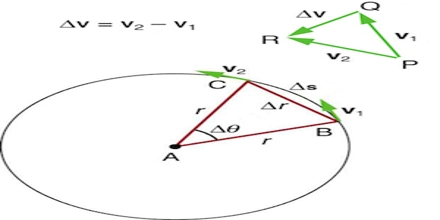Physics

# Centripetal AccelerationIn uniform circular motion, although the speed is constant, there is an acceleration because the direction of the velocity vector is always changing.  The acceleration of uniform circular motion is called centripetal acceleration. The direction of the centripetal acceleration is toward the center of the circle. The magnitude of the centripetal acceleration is constant for uniform circular motion. Acceleration is a change in velocity, either in its magnitude—i.e., speed—or in its direction, or both.